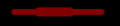# Quick question regarding impedance of microstrip.

#### phoenixyx

Joined Feb 8, 2017
18
Hi,

Just had a quick question regarding impedance of a microstrip as I am a bit curious on how this will work out in real life.

what happens if you change the width of a microstrip for only one place in the line as the example below. Lets say that all other factors/variables are the same for the full length.How will this affect the impedance, will you have to calculate impedances individually and add these in series? What would be the correct way to calculate the total impedance of this line? How will the wider part affect the circuit, will it lower the impedance for the full length, or will the thin line limit this.

Any tips on the mathematics for this?

best regards,
Viggo

#### cariban

Joined Aug 14, 2018
69
Hi,

Just had a quick question regarding impedance of a microstrip as I am a bit curious on how this will work out in real life.

what happens if you change the width of a microstrip for only one place in the line as the example below. Lets say that all other factors/variables are the same for the full length.
View attachment 165016

How will this affect the impedance, will you have to calculate impedances individually and add these in series? What would be the correct way to calculate the total impedance of this line? How will the wider part affect the circuit, will it lower the impedance for the full length, or will the thin line limit this.

Any tips on the mathematics for this?

best regards,
Viggo
The wider track will have lower characteristic impedance. Note that "characteristic impedance" means quite differently from impedance. In your case you may regard three independent sections are cascaded together. A two-port model with s-parameter is a better way to define the total properties. How this will affect your system? Just need to put the s-parameter in the system.

Of course there may be quick way to assess the impact to the system. If the length of the wider track is significantly shorter than the wavelength, you may model the total system as a uniform track with a small shunt capacitor in the middle.

#### loulou31

Joined Feb 23, 2018
15
First you have understand what is the impedance of a Line (quadriplole) . The good term is caracteristic impedance that has different meaning of impedance of a dipole. This is the reason why you cannot add the impedance of different lines in series of different length as you can do for discrete components : resistors, inductor, capacitor.

Jean-Louis